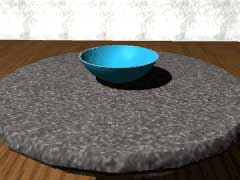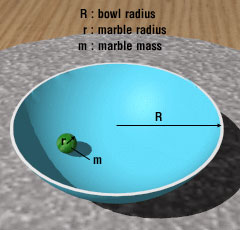Ch 10. Vibrations Multimedia Engineering Dynamics Free Vibs. Undamped Free Vibs. Damped Forced Vibration Energy Method
 Chapter - Particle - 1. General Motion 2. Force & Accel. 3. Energy 4. Momentum - Rigid Body - 5. General Motion 6. Force & Accel. 7. Energy 8. Momentum 9. 3-D Motion 10. Vibrations Appendix Basic Math Units Basic Equations Sections Search eBooks Dynamics Fluids Math Mechanics Statics Thermodynamics Author(s): Kurt Gramoll ©Kurt GramollDYNAMICS - CASE STUDY IntroductionProblem Graphic A marble in a spherical bowl oscillates with an apparently simple harmonic motion, however for large oscillations this motion becomes quite difficult to describe. What is known: marble radius r = 0.04 ft marble mass m = 0.003 slugs bowl radius R = 1 ftProblem Diagram Question What is the frequency of the marble's motion in the bowl for small oscillations? Approach Determine the kinetic energy and potential energy for the marble. Find the equation of motion by setting the time derivative of the total energy equal to zero. Solve the equation of motion.

Practice Homework and Test problems now available in the 'Eng Dynamics' mobile app
Includes over 400 problems with complete detailed solutions.
Available now at the Google Play Store and Apple App Store.